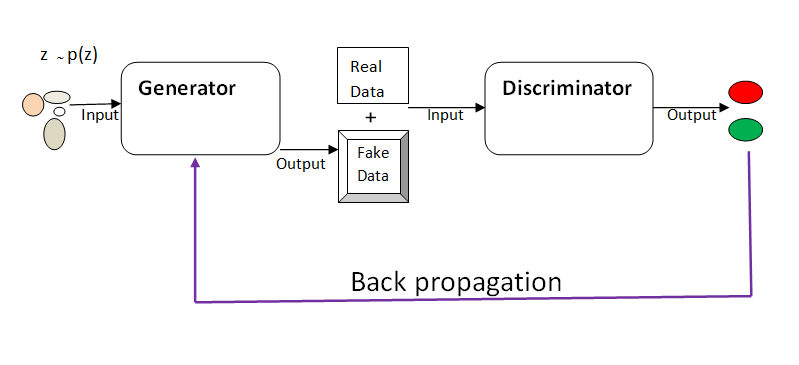$\color{red}{\text{ Draft: Work in Progress..... }}$

• GAN consist of two competing models striving to outdo each other: the Generator and Discriminator models.

• The Generator takes in random input and tries to generate real data (curves, images, texts, ).

• The Discriminator is a binary classifier. It takes, as its input, the fake data generated by the Generator, and the real dataset and learn to tell whether the data is real or fake.

• Through back propagation, the Generator updates its parameters using the output from the Discriminator.

• The two models are trained simultaneously with the aim that the continuous competition will help produce data that is indistinguishable from the real data.

Diagramatically…Given the objective function:

$\min_{G} \max_{D} F(G, D) = \log(D(x; \phi)) + \log(1- D( x^{'}; \phi))$

where:

• $z \sim p_{z}(z)$ is a random sample from distribution $p_{z}(z)$

• $x^{’} = G(z; )$
• $x$ is the real data from distribution $p_{d}(x)$

• Generator minimizes  by maximazing log $p(y= true|x^{’})$
• Discriminator maximizes by maximizing log $p(y= fake|x^{'})$ and $p(y= true|x)$

The models are trained simultaneously with the goal of obtaining a Nash equilibrium.

Share